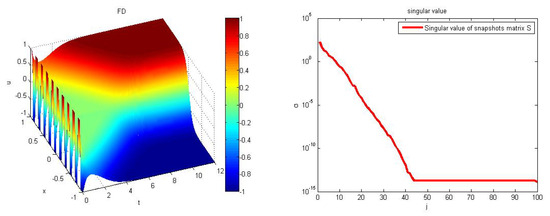Next Article in Journal
A Distributed Approach to the Evasion Problem
Previous Article in Journal
Local Comparison between Two Ninth Convergence Order Algorithms for Equations
Open AccessArticle

# An Application of a Modified Gappy Proper Orthogonal Decomposition on Complexity Reduction of Allen-Cahn Equation

byChutipong Dechanubeksa 1 andSaifon Chaturantabut 2,*1
Department of mathematics, Faculty of Science, King Mongkut’s University of Technology Thonburi, Bangkok 10140, Thailand
2
Department of mathematics and statistics, Faculty of Science and Technology, Thammasat University Rangsit Center, Pathumthani 12120, Thailand
*
Author to whom correspondence should be addressed.
Algorithms 2020, 13(6), 148; https://doi.org/10.3390/a13060148
Received: 26 May 2020 / Revised: 15 June 2020 / Accepted: 16 June 2020 / Published: 22 June 2020
This work considers model reduction techniques that can substantially decrease computational cost in simulating parmetrized Allen–Cahn equation. We first employ the proper orthogonal decomposition (POD) approach to reduce the number of unknowns in the full-order discretized system. Since POD cannot reduce the computational complexity of nonlinearity in Allen–Cahn equation, we also apply discrete empirical interpolation method (DEIM) to approximate the nonlinear term for a substantial reduction in overall simulation time. However, in general, the POD-DEIM approach is less accurate than the POD approach, since it further approximates the nonlinear term. To increase the accuracy of the POD-DEIM approach, this work introduces an extension of the DEIM approximation based on the concept of Gappy POD (GPOD), which is optimal in the least-squares sense. The POD-GPOD approach is tested and compared with the POD and POD-DEIM approaches on Allen–Cahn equation for both cases of fixed parameter value and varying parameter values. The modified GPOD approximation introduced in this work is demonstrated to improve accuracy of DEIM without sacrificing too much efficiency on the computational speedup, e.g., in one of our numerical tests, the POD-GPOD approach provides an approximate solution to the parmetrized Allen–Cahn equation 200 times faster than the full-order system with average error of order $O ( 10 − 4 )$ . The POD-GPOD approach is therefore shown to be a promising technique that compromises between the accuracy of POD approach and the efficiency of POD-DEIM approach. View Full-Text
Show FiguresFigure 1

MDPI and ACS Style

Dechanubeksa, C.; Chaturantabut, S. An Application of a Modified Gappy Proper Orthogonal Decomposition on Complexity Reduction of Allen-Cahn Equation. Algorithms 2020, 13, 148.